# Proactive Compaction

## for the Linux kernel

This feature has now been accepted and merged in the upstream kernel and will be part of kernel release 5.9. This post has been updated to match the upstream version of this feature.

In my previous post, I described how on-demand compaction scheme hurts hugepage allocation latencies on Linux. To improve the situation, I have been working on Proactive Compaction for the Linux kernel, which tries to reduce higher-order allocation latencies by compacting memory in the background.

# Design

The Linux kernel uses per-NUMA-node threads called kcompactdN (where N is the NUMA node ID), which are responsible for compacting their corresponding NUMA node. We reuse these per-node threads to do proactive compaction: each of these threads now periodically calculates per-node “proactive compaction score”, and checks it against a threshold. This threshold is derived from a new sysctl called vm.compaction_proactiveness, which accepts values in [0, 100] with a default value of 20.

The Linux kernel divides a node’s memory into “zones”, typically ZONE_DMA32, ZONE_DMA, and ZONE_NORMAL, where the last one holds the most memory while others are special purpose zones and are typically manage small amounts of total RAM.

For proactive compaction purposes, let’s first define a zone’s score ($S_z$):

$$S_z = \frac{N_z}{N_n} * extfrag(zone, HUGETLB\_PAGE\_ORDER)$$

where:

• $N_z$ is the total number of pages in the zone.
• $N_n$ is the total number of pages in the zone’s parent node.
• $extfrag(zone, HUGETLB\_PAGE\_ORDER)$ is the external fragmentation with-respect-to the hugepage order in this zone. In general, to calculate external fragmentation wrt any order:

$$extfrag(zone, order) = \frac{F_z - H_z}{F_z} * 100$$

where:

• $F_z$ is the total number of free pages in the zone.
• $H_z$ is the number of free pages available in blocks of size >= $2^{order}$.

This per-zone score value is in range [0, 100], and is defined as 0 when $F_z=0$. The reason for calculating the per-zone score this way is to avoid wasting time trying to compact special zones like ZONE_DMA32 and focus on zones like ZONE_NORMAL, which manage most of the memory ($N_z \approx N_n$). For smaller zones ($N_z \ll N_n$), the score $\to$ 0, and thus will never cross the (low) threshold value.

Next, a node’s score ($S_n$) is the sum of its zone’s scores:

$$S_n = \sum S_z$$

This per-node score value is in range [0, 100].

Finally, here’s how we define low ($T_l$) and high ($T_h$) thresholds, against which these scores are compared:

$$T_l = 100 - vm.compaction\_proactiveness$$

$$T_h = min(10 + T_l, 100)$$

These thresholds are in range [0, 100].

With per-node and per-zone scores and thresholds defined, let’s see the updated flow for per-node kcompactd threads with proactive compaction: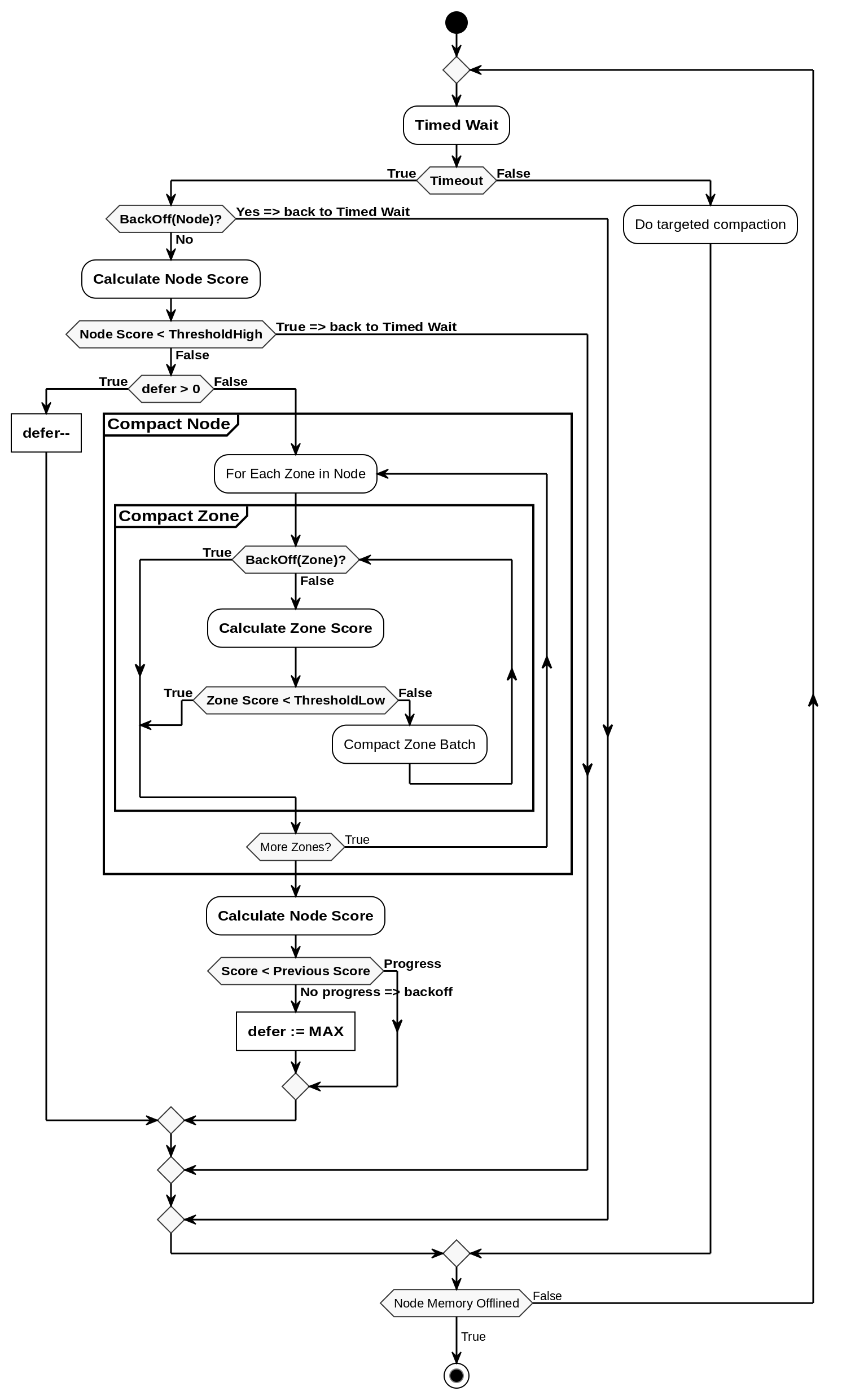Figure 1: Activity flow diagram of a kcompactdN thread with proactive compaction. New elements added to the usual flow are marked as bold.

Note that we use backoff at both node level: BackOff(Node) and at zone level: BackOff(Zone). In its current form, we define backoff conditions as:

BackOff(Node)

• Don’t start proactive compaction when kswapdN thread is active on node N. This backoff is to prevent kswapd and kcompactd threads from interfering. Typically we want to run compaction after a round of reclaim to fill in the holes created during the reclaim process.

BackOff(Zone)

• Compaction acquires a very coarse grain, node level lock (pg_data_t.lock). When compaction is done in a proactive context, we check if we had to contend for this lock. If yes, we back off to give other contenders a better chance to get the lock. The goal is to avoid hurting non-background, latency-sensitive contexts.

# Performance

System Configuration: x86_64, 1T RAM, 80 CPUs, 2 NUMA nodes.

Kernel: 5.6.0-rc4

Before starting any benchmarks, the system memory was fragmented from a userspace program called “frag”, which I have described in detail in my previous post. In summary, it allocates almost 1T of memory and then, for every 2M aligned region (default hugepage size on x86_64), frees $\frac{3}{4}$ th of base (4K) pages. This allocation/free pattern leaves no hugepages directly available for allocation. Thus, every hugepage allocation now hits the slow path where it has to through direct compaction process.

Such a fragmented memory state emulates a typical, long-running server (or even desktop) machine. Test programs show significant reduction in hugepage allocation latencies with proactive compaction on such systems.

## 1. Kernel hugepage allocation latencies

The first test program is a kernel driver which tries to allocate as much memory as possible as hugepages using __alloc_pages(GFP_KERNEL). It measures the latency of each allocation, and it stops on the first allocation failure. With vanilla kernel, all these allocation requests hit direct compaction (since we have fragmented the memory as described above). However, with this patch applied, memory is compacted in background as the driver allocates hugepages in parallel, reducing allocation latencies.

Here are allocation latencies (in $\mu s$) as reported by this test driver:

• With vanilla 5.6.0-rc4
StatLatency
$count$$383859 min$$1.0$
$max$$158238.0 \mu$$21746.41$
$\sigma$$9278.36 Total 2M hugepages allocated = 383859 (749G worth of hugepages out of 762G total free => 98% of free memory could be allocated as hugepages). In terms of percentiles: PercentileLatency 5$$7894$
$10$$9496 25$$12561$
$30$$15295 40$$18244$
$50$$21229 60$$27556$
$75$$30147 80$$31047$
$90$$32859 95$$33799$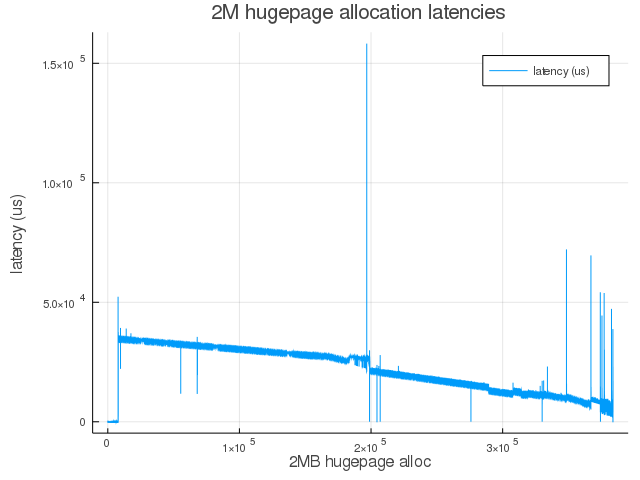Figure 2: Hugepage allocation latencies from kernel space under fragmented memory conditions with vanilla 5.6.0-rc4 kernel.

• With 5.6.0-rc4 + this patch, with vm.compaction_proactiveness=20
sudo sysctl -w vm.compaction_proactiveness=20

StatLatency
$count$$384105 min$$2.0$
$max$$300683.0 \mu$$150.9$
$\sigma$$1063.6 Total 2M hugepages allocated = 384105 (750G worth of hugepages out of 762G total free => 98% of free memory could be allocated as hugepages) In terms of percentiles: PercentileLatency 5$$2$
$10$$2 25$$3$
$30$$3 40$$3$
$50$$4 60$$4$
$75$$4 80$$4$
$90$$5 95$$429$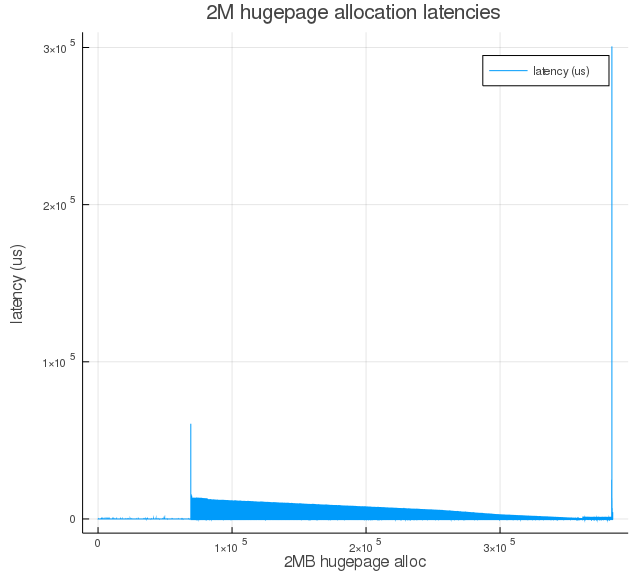Figure 3a: Hugepage allocation latencies from kernel space under fragmented memory conditions with 5.6.0-rc4 kernel + proactive compaction patch and proactiveness=20. The initial run of near-zero latencies is due to already available hugepages. Once they are consumed, proactive compaction kicks in to keep latencies low.

For some more insight into proactive compaction behavior, here are plots with increasing values on proactiveness: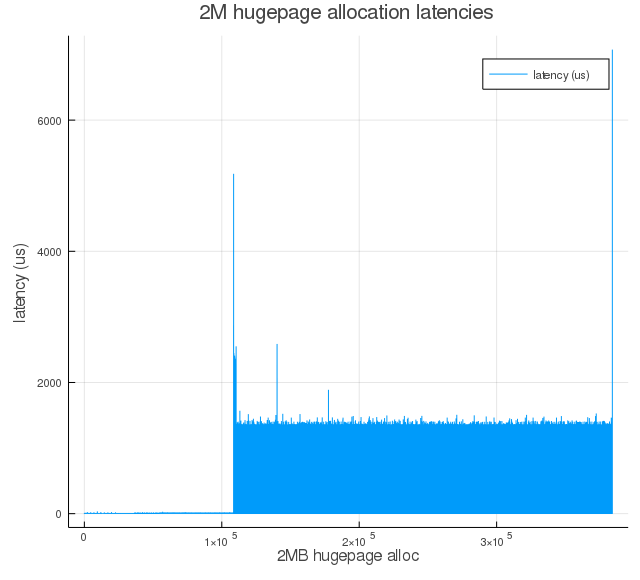Figure 3b: Same as Figure 3a, but with proactiveness=30.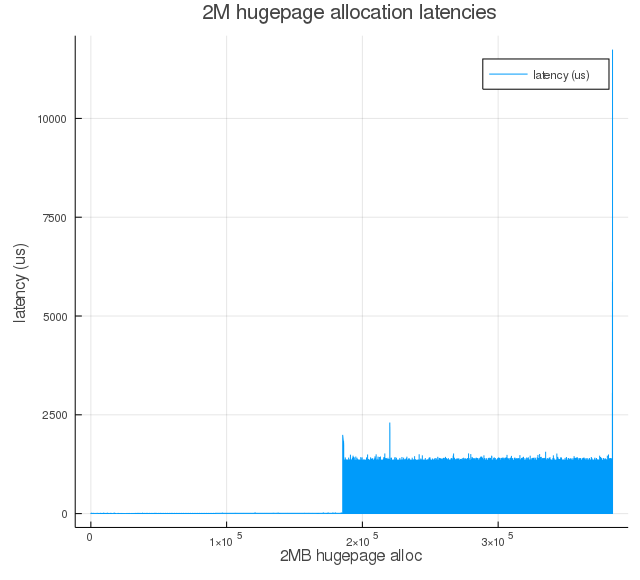Figure 3c: Same as Figure 3a, but with proactiveness=50.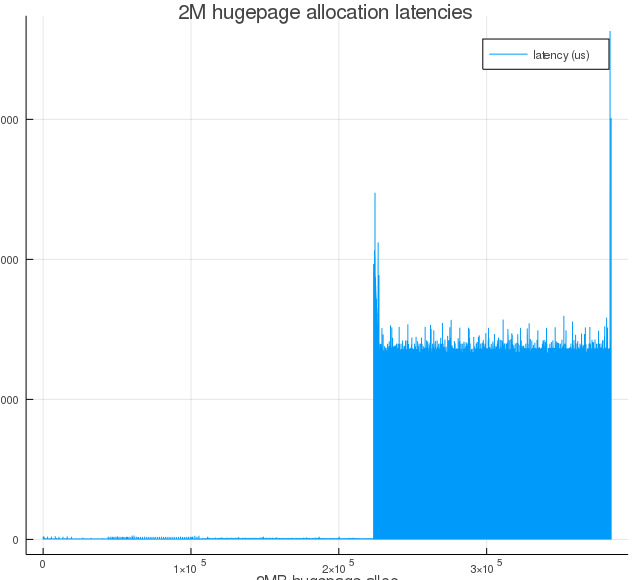Figure 3d: Same as Figure 3a, but with proactiveness=60.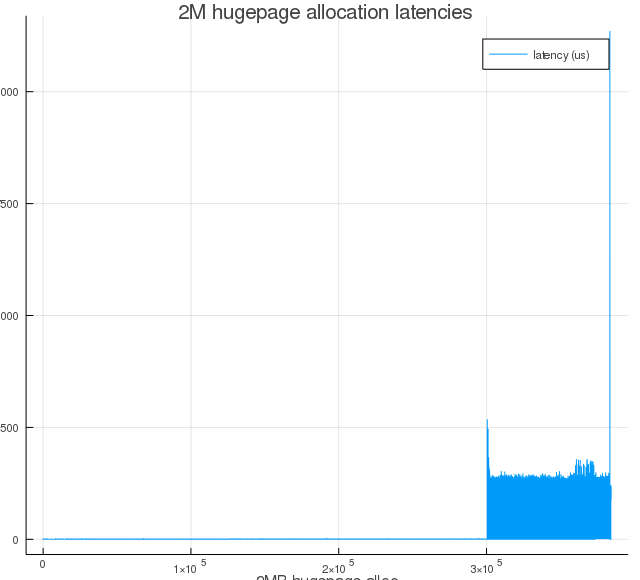Figure 3e: Same as Figure 3a, but with proactiveness=80.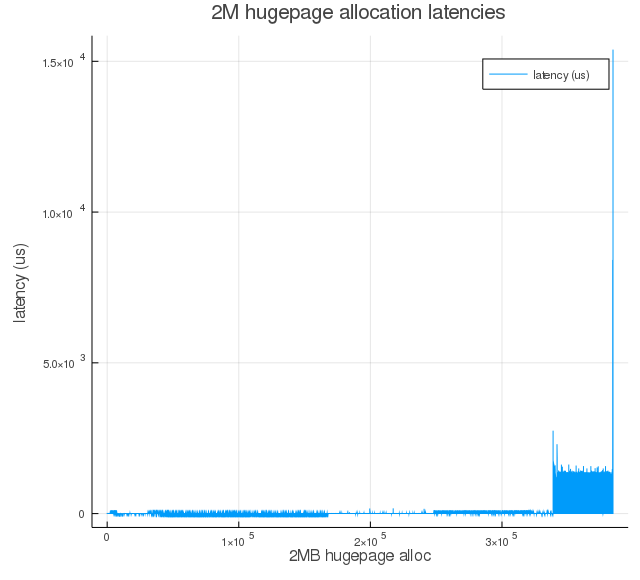Figure 3f: Same as Figure 3a, but with proactiveness=90.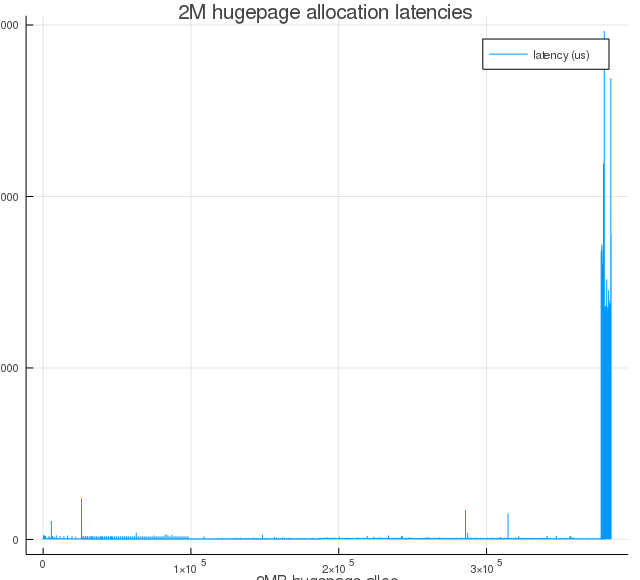Figure 3g: Same as Figure 3a, but with proactiveness=100.

Notes

For proactiveness=20, The mean hugepage allocation latency dropped from $21746.41 \mu s$ to $150 \mu s$. In terms of percentiles, 95-percentile latency went from $32859 \mu$s to $429 \mu s$.

As we increase proactiveness value, we see larger runs of near-zero latencies. That is because of proactive compaction bringing down the node score proportionally (see, $T_l$ and $T_h$ equations), and this initial round of compaction happened between the time when memory was fragmented and when the kernel driver was triggered to start allocating hugepages.

## 2. Java heap allocation

In this test, we start a Java process with a heap size set to 700G and request the heap to be allocated with THP hugepages and to touch each heap page post allocation, which ensures that the process doesn’t get the special zero-hugepage. We also set THP to madvise to allow hugepage backing of this heap (internally, JVM does madvise(MADV_HUGEPAGE, ... for the heap area when started with parameters, as shown below).

echo madvise | sudo tee /sys/kernel/mm/transparent_hugepage/enabled
echo madvise | sudo tee /sys/kernel/mm/transparent_hugepage/defrag


Before we start this Java process, we fragment the system memory using the same method as for (1). With the system memory in fragmented state, launch the Java process as follows to allocate 700G of hugepages-backed heap area:

/usr/bin/time java -Xms700G -Xmx700G -XX:+UseTransparentHugePages -XX:+AlwaysPreTouch

• With vanilla 5.6.0-rc4

17.39user 1666.48system 27:37.89elapsed

• With 5.6.0-rc4 + this patch, with vm.compaction_proactiveness=20

8.35user 194.58system 3:19.62elapsed

Elapsed time remains around 3:15, as we further increase the proactiveness value.

Note that proactive compaction happens throughout the runtime of these benchmarks. The situation of one-time compaction, sufficient to supply hugepages for following allocation stream, can probably happen for more extreme proactiveness values, like 80 or 90. That’s why I chose a smaller value like 20 to make sure proactive compaction goes on in parallel with the allocating program for more realistic numbers.

Here is a plot which shows how the per-node compaction score varies as this java benchmark progresses: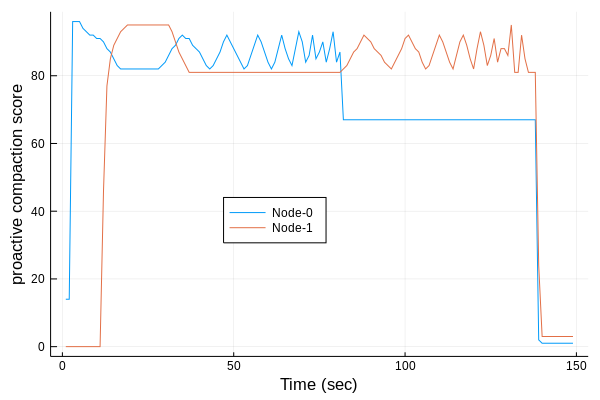Figure 4: Changes in proactive compaction score for Node-0 and Node-1 as Java heap allocation benchmark is running. Each time the score exceeds the high threshold of 90, proactive compaction is triggered and runs till the score reaches the low threshold of 80. These thresholds are based on a proactiveness value of 20.

In the run corresponding to Figure 4, the Java benchmark finished in just over 2 mins with vm.compaction_proactiveness=20, but that’s just run-to-run variation. The interesting part is how per-node proactive compaction score zig-zags between high and low score thresholds (low=80, high=90 when vm.compaction_proactiveness=20). First, the node-0 was actively compacted, and when it reached its capacity, allocations were satisfied from node-1, which was then proactively compacted to keep the score within thresholds. During times of active compaction, kcompactd0/1 threads consumed 100% of one of the CPUs.

Notes

Time to allocate 700G Java heap under fragmented memory conditions came down from 27mins 37secs to 3min 19secs. We also saw how proactive compaction runs in parallel with the workload to keep the score within thresholds (derived from user-provided vm.compaction_proactiveness value).

# Backoff/Defer behavior

Compaction is an expensive operation. For each page, it has do unmap, copy, and remap operations which can cause random pauses, or increases access latencies (due to TLB misses) in any of the running applications. This system-wide impact must be taken into account and balanced with the benefits of a well-compacted memory state (reduced hugepage allocated latencies).

In the above benchmarks, we fragmented the memory from userspace using “private, anonymous” pages. These kinds of pages are easy to move around and thus can be effectively compacted. But what if the memory is filled with “unmovable” pages like kernel allocations (dentry cache, etc.) spread randomly throughout the physical memory? In such cases, we cannot really compact memory, and thus we must back off any proactive compaction attempts.

To simulate such a memory state, I created a kernel driver that allocates almost all memory as hugepages followed by freeing the first 3/4-th of each hugepage.

I set vm.compaction_proactiveness=40, and as expected, each time the system attempts proactive compaction, it could not bring down the score for any node. So, it was deferred the maximum number of times with each failed attempt. With the current design, the wait between subsequent tries in case of maximum number of defers is ~30secs, and thus, kcompactd thread’s CPU usage remained almost zero.

# Summary

Performance numbers with proactive compaction show a significant reduction in hugepage allocation latencies under fragmented memory conditions, together with an effective backoff/defer strategy when dealing with memory states where compaction cannot help.

This feature has been accepted and merged in the upstream kernel and will be part of kernel 5.9.